## A random sample of 30 patties that were inspected over the course of the last week revealed that the average weight was 95.0 grams. The stan

Question

A random sample of 30 patties that were inspected over the course of the last week revealed that the average weight was 95.0 grams. The standard deviation was 0.25 grams. What percentage of the deliveries is likely to be outside the specification limits (outside the interval of [94.5, 95.5])

in progress 0
2 weeks 2021-09-04T20:51:25+00:00 1 Answers 0 views 0

4.56% of the deliveries are likely to be outside the specification limits.

Step-by-step explanation:

Normal Probability Distribution:

Problems of normal distributions can be solved using the z-score formula.

In a set with mean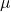and standard deviation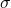, the z-score of a measure X is given by: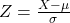The Z-score measures how many standard deviations the measure is from the mean. After finding the Z-score, we look at the z-score table and find the p-value associated with this z-score. This p-value is the probability that the value of the measure is smaller than X, that is, the percentile of X. Subtracting 1 by the p-value, we get the probability that the value of the measure is greater than X.

The average weight was 95.0 grams. The standard deviation was 0.25 grams.

This means that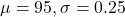What percentage of the deliveries is likely to be outside the specification limits (outside the interval of [94.5, 95.5])?

Less than 94.5, or more than 95.5. Since the normal distribution is symmetric, these probabilities are the same, so we can find one of them and multiply by two.

The probability that it is less than 94.5 is the p-value of Z when X = 94.5. So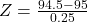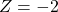has a p-value of 0.0228

2*0.0228 = 0.0456

0.0456*100% = 4.56%

4.56% of the deliveries are likely to be outside the specification limits.